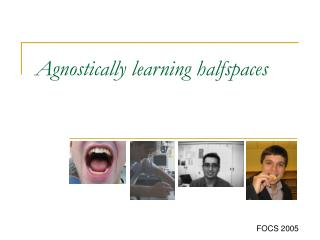DownloadDownload PresentationAgnostically learning halfspaces

# Agnostically learning halfspaces

Download Presentation## Agnostically learning halfspaces

- - - - - - - - - - - - - - - - - - - - - - - - - - - E N D - - - - - - - - - - - - - - - - - - - - - - - - - - -
##### Presentation Transcript

1. Agnostically learning halfspaces FOCS 2005

2.  w.h.p. h: X!{0,1} poly(1/) samples P [h(x)y] · opt +  P[f*(x)y] arbitrary dist. over (x,y) 2X £ {0,1} f* = argminf2F P [f(x)y] L. Sellie Agnostic learning Set X, F class of functionsf: X!{0,1}. Efficient Agnostic Learner

3. w.h.p. h: Xn!{0,1} P [h(x)y] · opt +  P[f*(x)y] arbitrary dist. over (x,y) 2X £ {0,1} f* = argminf2F P [f(x)y] L. Sellie Agnostic learning Set XnµRn, Fn class of functionsf: Xn!{0,1}. n Efficient Agnostic Learner  poly(n,1/) samples

4. w.h.p. h: Xn!{0,1} P[f*(x)y] arbitrary dist. over (x,y) 2X £ {0,1} f* = argminf2F P [f(x)y] L. Sellie Agnostic learning Set XnµRn, Fn class of functionsf: Xn!{0,1}. n Efficient Agnostic Learner  poly(n,1/) samples P [h(x)y] · opt +  in PAC model, P [f*(x)y] = 0

5. P[f*(x)y] h f* argminf2F P[f(x)y] Agnostic learning of halfspaces Fn = { f(x)=I(w¢x¸)| w2Rn, 2R }. h: Rn!{0,1} P [h(x)y] · opt + 

6. P[f*(x)y] h f* Agnostic learning of halfspaces Fn = { f(x)=I(w¢x¸)| w2Rn, 2R }. h: Rn!{0,1} P [h(x)y] · opt +  Special case: junctions, e.g.,f(x) = x1 Ç x3 = I(x1 + x3 ¸ 1) • Efficient agnostic-learn junctions ) PAC-learn DNF • NP-hard to properly agnostic learn

7. P[f*(x)y] f* Agnostic learning of halfspaces PAC learning halfspaces solved by LP Fn = { f(x)=I(w¢x¸)| w2Rn, 2R }. h: Rn!{0,1} P [h(x)y] · opt + 

8. P[f*(x)y] h f* Agnostic learning of halfspaces PAC learning halfspaces with indep./random noise solved by: Fn = { f(x)=I(w¢x¸)| w2Rn, 2R }. h: Rn!{0,1} P [h(x)y] · opt + 

9. h f* Agnostic learning of halfspaces Fn = { f(x)=I(w¢x¸)| w2Rn, 2R }. h: Rn!{0,1} P [h(x)y] · opt +  minf2FnP[f(x)y] Equivalently, f*=“truth” with adversarial noise

10. nO(-4) Theorem 1: (w.h.p.) Our alg. outputs h: Rn!{0,1} with P[h(x)  y] · opt + , in time poly(n) (8 const >0), as long as draws x 2 Rn from: • Log-concave distribution, e.g.: uniform over convex set, exponential e-|x|, normal • Uniform over {-1,1}nor Sn-1={x2Rn| |x|=1} • …

11. 2. Low-degree Fourier algorithm of • Chose , where • Outputh(x) = I(p(x)¸½) time nO(d) 1. L1polynomial regression algorithm ¼ minimizedeg(p)·d E [|p(x)-y|] • Given: d>0,(x1,y1),…,(xm,ym) 2Rn£ {0,1} • Find deg-d p(x) to minimize: • Pick 2 [0,1] at random, output h(x) = I(p(x)¸) multivariate time nO(d) ¼ minimizedeg(p)·d E [(p(x)-y)2] (requires x uniform from {-1,1}n) y x

12. ·p lemma of : alg’s error· ½ - (½ - opt)2 + & Sellie 1. L1polynomial regression algorithm ¼ minimizedeg(p)·d E [|p(x)-y|] • Given: d>0,(x1,y1),…,(xm,ym) 2Rn£ {0,1} • Find deg-d p(x) to minimize: • Pick 2 [0,1] at random, output h(x) = I(p(x)¸) multivariate lemma: alg’s error · opt + mindeg(q)·dE [|f*(x)-q(x)|] 2. Low-degree Fourier algorithm of • Chose , where • Outputh(x) = I(p(x)¸½) ¼ minimizedeg(p)·d E [(p(x)-y)2] (requires x uniform from {-1,1}n) time nO(d) lemma: alg’s error·8(opt + mindeg(q)·dE [(f*(x)-q(x))2]) = e y x

13. Useful properties of logconcave dist’s: projection is logconcave, …, Approx degree is dimension-free for halfspaces q(x) ¼I(x ¸ 0) degree d=10 q(w¢x) ¼I(w¢x¸0) degree d=10

14. Hey, I’ve used Hermite (pronounced air-meet) polynomials many times. Approximating I(x ¸) (1 dimension) • Bound mindeg(q)·dE[(q(x) – I(x ¸))2] • Continuous distributions: orthogonal polynomials • Normal: Hermite polynomials • Logconcave (e-|x|/2 suffices): new polynomials • Uniform on sphere: Gegenbauer polynomials • Uniform on hypercube: Fourier <f,g> = E[f(x)g(x)]

15. Theorem 2: junctions (e.g., x1Æ x11Æ x17) • For arbitrary over {0,1}n£{0,1} the polynomial regression algorithm with d=O(n1/2log(1/)) (time -O*(n½)) outputs h with P[h(x)y] · opt +  Follows from previous lemmas +

16. Assume (x,y) = (1-) (x,f*(x)) +  (arbitrary (x,y)): • We get: error · O(n1/4 log(n/))  + using Rankin’s second bound uniform 2 Sn-1 How far can we get in poly(n,1/) time? Assume draws x uniform from: Sn-1 = { x2Rn| |x|=1} • Perceptron algorithm: error · O(pn) opt +  • We show: simple averaging algorithm of achieves error · O(log(1/opt)) opt + 

17. Half-space conclusions & future work • L1 poly reg: natural extension of Fourier learning • Works for non-uniform/arbitrary distributions • Tolerates agnostic noise • Works on both continuous and discrete problems • Future work • Work on all distributions (not just logconcave/uniform {-1,1}n) • opt +  using poly(n,1/) algorithm (we have poly(n) for fixed , and trivial: poly() for fixed n) • Other interesting classes of functions